【阅读时间】10min 3326words
【阅读内容】通过构造知识联想链条直观例子回答什么是泰勒级数，为什么需要泰勒级数，泰勒级数干了什么，如何记忆这个公式

For me, mathematics is a collection of examples; a theorem is a statement about a collection of examples and the purpose of proving theorems is to classify and explain the examples

John B. Conway

# 什么是泰勒公式

## 基本定义

\begin{align} f(x)_{Taylor} &= \sum_{n=0}^{\infty} \frac{f^{(n)}(a)}{n!} \times (x - a)^n \\ &= f(a) + \frac{f'(a)}{1!}(x-a) + \frac{f^{(2)}(a)}{2!}(x-a)^2+ \cdots + \frac{f^{(n)}(a)}{n!}(x-a)^n + R_n(x) \end{align}

$f^{(n)}(a)$ 表示 $f(x)$ 在第 $n$ 阶导数的表达式，带入一个值 $a$ 计算后得到的结果（注意，它是个值）
$\frac{1}{n!}$ 是一个系数（一个值），每一项都不同，第一项 $\frac{1}{1}$，第二项 $\frac{1}{2!}$ …… 依此类推
$(x-a)^n$ 是一个以 $x$ 为自变量的表达式

$R_n(x)$ 是泰勒公式的余项，是 $(x-a)^n$ 的高阶无穷小（此处先不解释，听起来很牛逼，但是跟随例子看完就发现并没有什么玄乎的）

## 联想链条

【公式】 ＜什么公式？＞➜ 【多项式】（Polynomials），把多项式的一般形式写出来，这应该是非常容易理解的概念，即指数不仅仅为2的抛物线的组合

$$P_{n}(x) = \sum_{i = 0}^{n} c_ix^i = c_0 + c_1x + c_2x^2 + \cdots + c_nx^n$$

【泰勒】＜谐音“太乐” ≈ 如果所有小数都能近似成整数那不是太快乐了？＞ ➜ 近似，获得一个直观理解

• 泰勒公式通过把【任意函数表达式】转换（重写）为【多项式】形式，是一种极其强大的函数近似工具

• 多项式非常【友好】，三易，易计算，易求导，易积分
• 几何感觉和计算感觉都很直观，如抛物线和几次方就是底数自己乘自己乘几次

# 怎样理解泰勒公式

（只有三项是为图个方便）去近似余弦函数 $f(x) = cos(x)$ ，【近似过程】参考下面的动图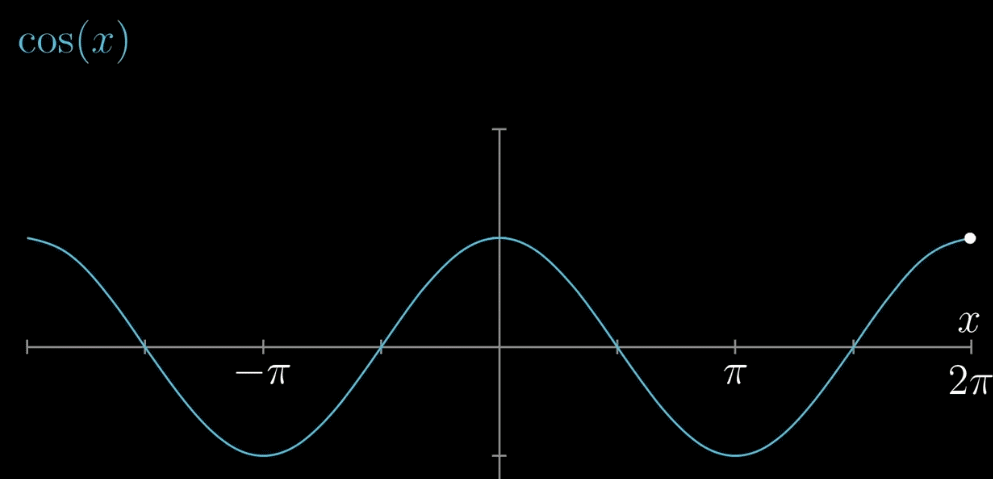## 函数式角度

### 近似过程

• 【确定 $c_0$】$x=0$ 带入公式，令 $cos(x) = 1$ ，同理对 $P(x)$ 可以得到 $c_0=1$• 【确定 $c_1$】容易观察到，如果对 $P(x)$ 求导就可以把 $c_1$ 前的自变量去掉。并且，$x=0$ 处 $P(x)$ 已经固定为1，为了更进一步的相似，如果我们让 $x=0$ 处的 $f(x)$ 和 $P(x)$ 的切线斜率也相同不就更近似了？（两种思考模式我觉得都可以） 求导之后可以的到 $c_1 = 0$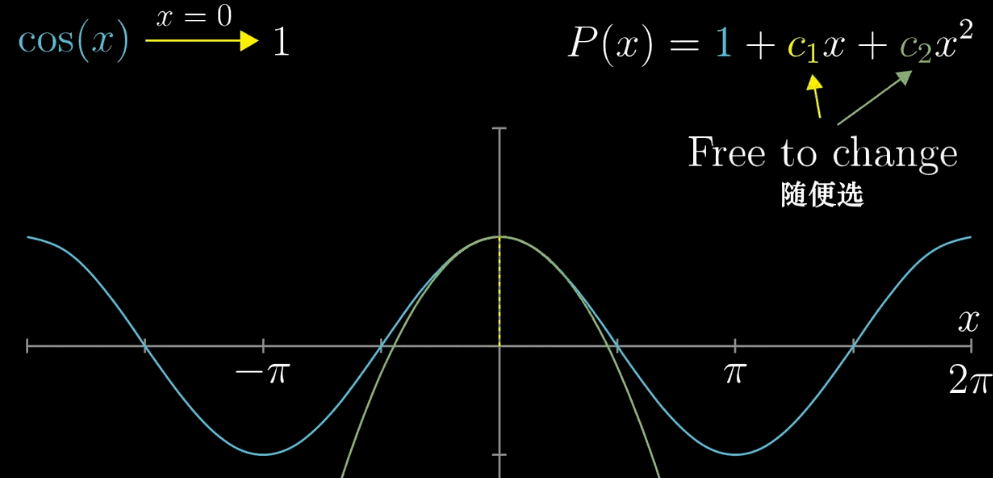• 【确定 $c_2$】现在我们已经确定两个值，那么绿色曲线就只能如下图一样移动（固定了 $x=0$ 的函数值和 $x=0$ 处的斜率 ），为了更接近相似的目标，我们希望斜率在变化的过程中速度也是近似的（滑动的白色和黄色直线）。求二次导数，斜率的变化率相等，确定 $c_2 = -\frac{1}{2}$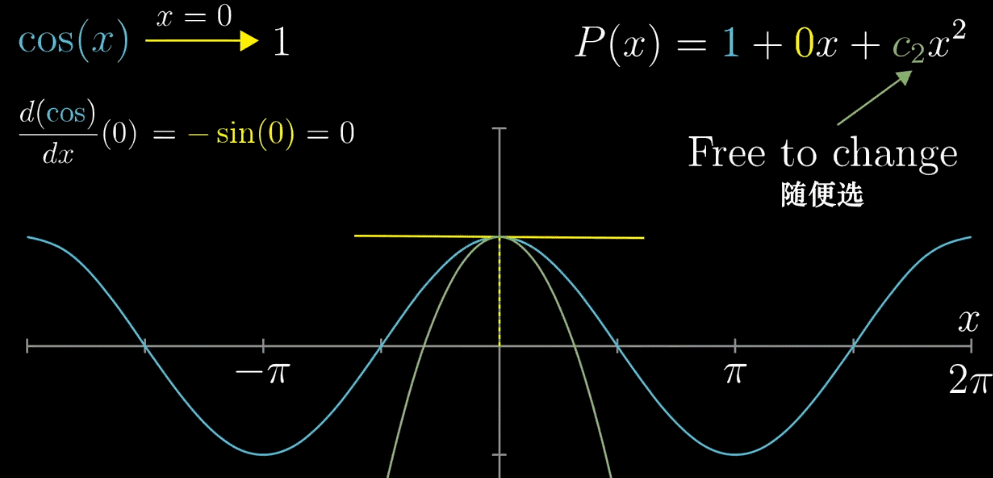### 为什么使用多项式来近似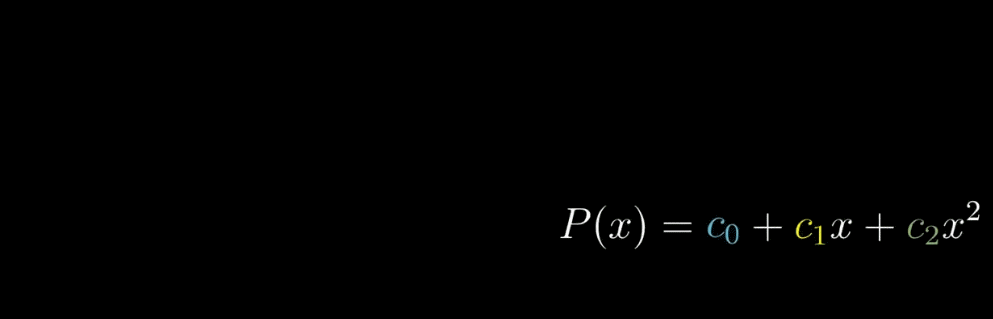$c_0$ 确保了 $x=0$ 时相等，$c_1$ 确保了 $x=0$ 时的斜率相等，$c_2$ 确保了 $x=0$ 时斜率的变化率相等，或者说，随着多项式幂次变高，这种近似就越精确

### 为什么有个系数 $\frac{1}{n!}$### 函数角度总结

• 首先，对于 $cos(x)$ 这个具体例子，把 $x=0$ 位置的多阶导数求出，再使用多项式进行近似，使用的项越多，得到的近似就越准确，参看下面动图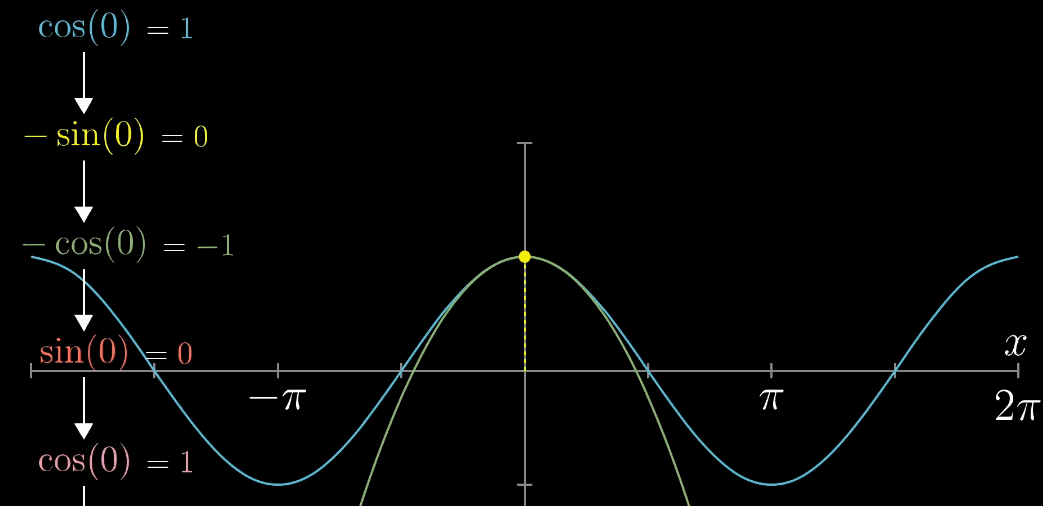• 推广到一般函数 $f(x)$ ,下列动图描述了随着项的增加，$x=0$ 附近的越来越准确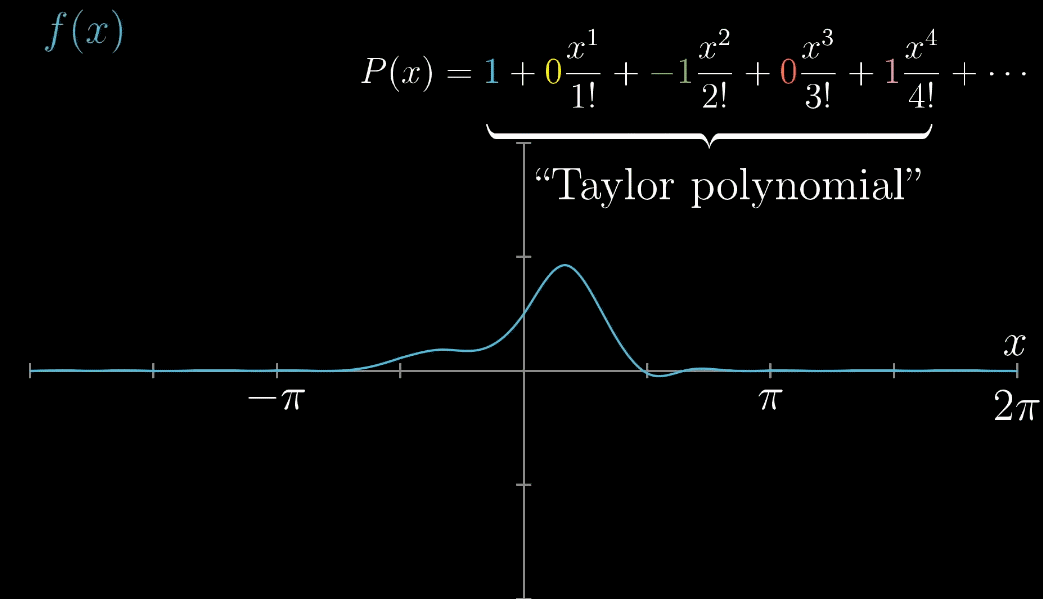• 最后，推广到 $x=a$ 的情形，完全推导出泰勒展开式的一般形式，如下列动图所示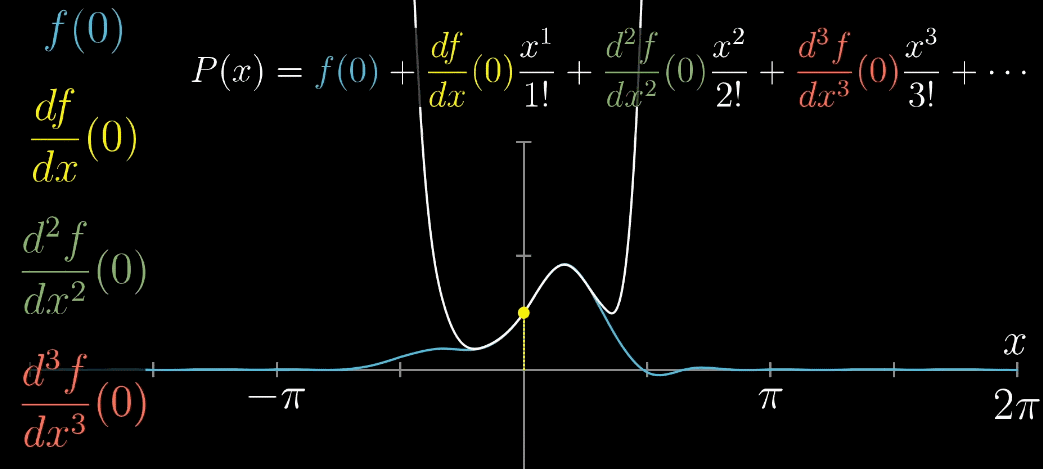## 几何角度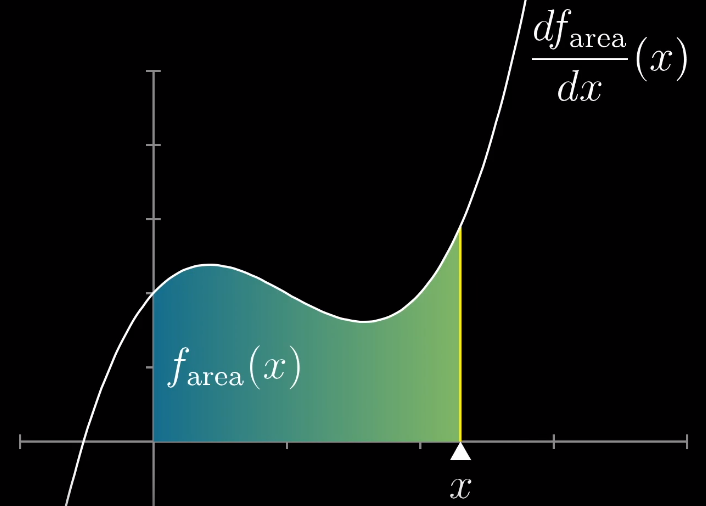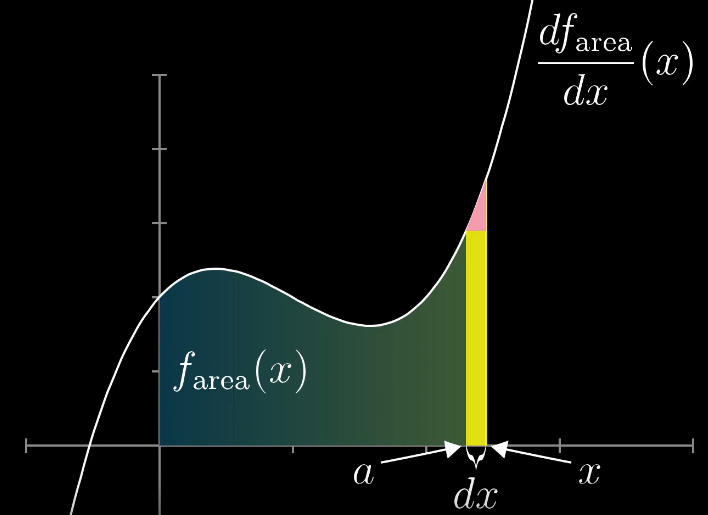• 【黄色矩形】底边为 $x-a$ ；高为 $\frac{df_{area}}{dx}(a)$ ；
• 【红色三角形】底边为 $x-a$；高的计算稍微麻烦，首先，斜边的斜率是 $\frac{df_{area}}{dx}(x)$ 函数的导数在 $x=a$ 时的函数值（算斜率，求导数即可），而斜率 $k = \frac{y}{x}$ ，所以得到高为 $\frac{d^2f_{area}}{dx^2}(a) \times (x-a)$ （前部分是斜率，后半部分是 $x$ ，需要求的是 $y$ 也是高）
• 【计算总面积】如下图和公式所示
$$f_{area}(x) \approx f(a) + \frac{df_{area}}{dx}(a)(x-a) + \frac{1}{2} \frac{d^2f_{area}}{dx^2}(a)(x-a)^2$$# 泰勒级数

## 收敛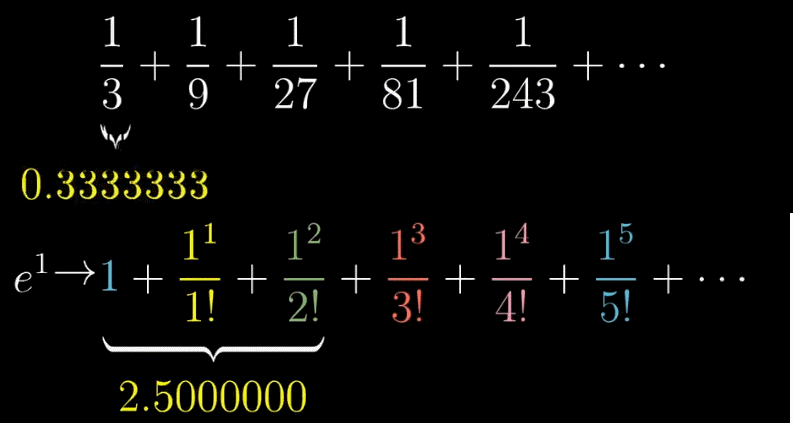## 发散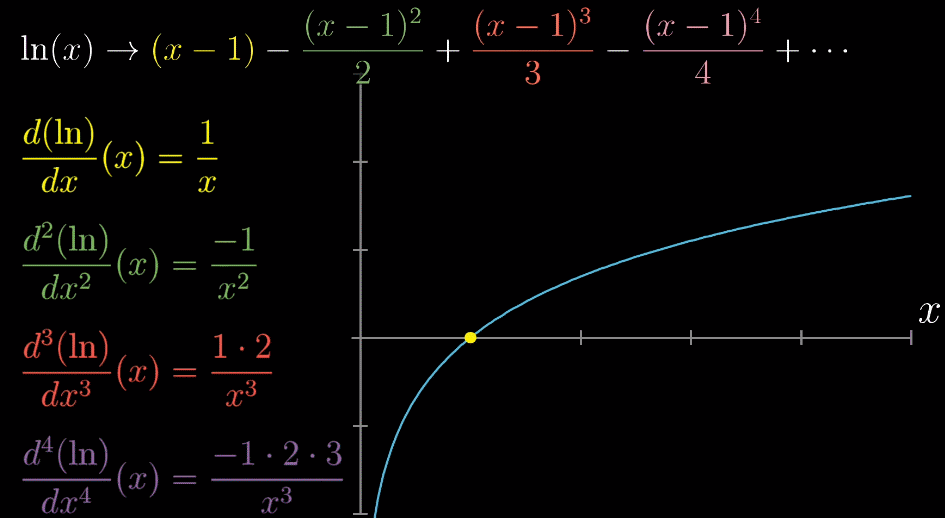# 总结

• 泰勒公式干了一件什么事？

• 泰勒公式的导数项如何推倒出来的？

• 泰勒公式如何记永远不会忘？

$$f(x) = \sum_{n=0}^\infty \frac{f^{(n)}(a)}{n!} (x-a)^n$$